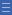# Using tens

Using tens

Students who are in the early stages of learning about place value need to understand the power of grouping ones into sets of 10. They also need to know the way tens and ones groupings are represented in written numerals.

To develop the use of place value as a tool for calculation, begin with the link between the addition and subtraction of ones, and then the same operations with groups of 10.

For example, 4 + 3 = 7 so 40 + 30 = 70.

Use 'story shells' to put the operations in context.

Initially students may want to skip count in tens (e.g. 40, 50, 60, 70). Encourage them to anticipate the answer by using facts they know before they count. You can watch the video Adding Tens.

Include addition and subtraction problems that bridge 100 to develop the idea of nested place value (i.e. tens are nested in hundreds, ones are nested in tens).

The thinking needed to bridge tens with units of one can be extended to addition and subtraction of tens.

For example, if 9 + 6 = 15 then 90 + 60 = 150.

Additions and subtractions of this type can be practised using the learning objects L92 The part-adder: make your own hard sums  and L98 The take-away bar: make your own hard subtractions.

Yes

Yes

Name Class SectionYear 2: Solve simple addition and subtraction problems using a range of efficient mental and written strategies Infobox 3Source Infobox 3